## Friday, October 23, 2015

### Node, edge, & tip labels for plotted cophylo objects

A recent blog comment asked if there was some way to 'maintain support values' (i.e., include node, edge, or tip labels) for a plotted `"cophylo"` object produced by phytools.

This is not quite as straightforward as it might seem because, unlike for a single plotted tree, there are two sets of node, two sets of tips, two sets of edges etc.

My solution was as follows:

First, I have the internally used tree plotting function, `phylogram`, create an environmental variable `"last_plot.phylo"`, as do other tree plotting methods such as `plot.phylo` and `plotSimmap`.

Next, I had the S3 `plot` method for objects of class `"cophylo"` pull each of these two `"last_plot.phylo"` objects from their environment (one for each tree plotted) and create a new environmental variable, `"last_plot.cophylo"`, in the same environment, consisting of a simple list of these two objects.

Finally, I created a series of wrappers around `nodelabels`, `tiplabels`, and `edgelabels`, which take as input which tree is to have labels added to it, and then sets the corresponding element of `"last_plot.cophylo"` to `"last_plot.phylo"` so that `nodelabels` etc. can be called internally.

Here's a demo using the following two trees which have matching tree labels. (Remember, we can use non-matching labels too if we are prepared to supply an association matrix.)

``````library(phytools)
packageVersion("phytools")
``````
``````##  '0.5.4'
``````
``````par(mfrow=c(1,2))
plotTree(t1)
plotTree(t2)
``````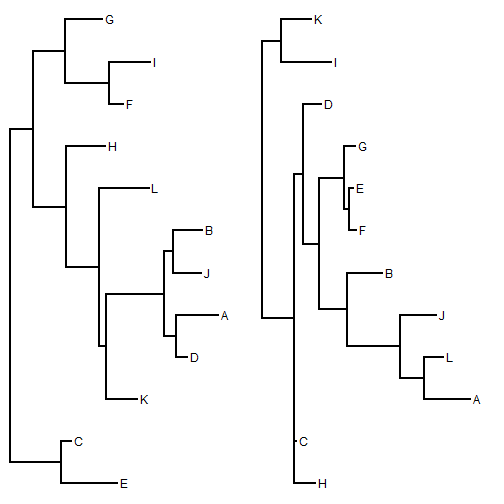Now, let's create an object of class `"cophylo"` and then try each of our new labeling methods:

``````obj<-cophylo(t1,t2)
``````
``````## Rotating nodes to optimize matching...
## Done.
``````
``````plot(obj)
nodelabels.cophylo() ## left side
nodelabels.cophylo(which="right")
``````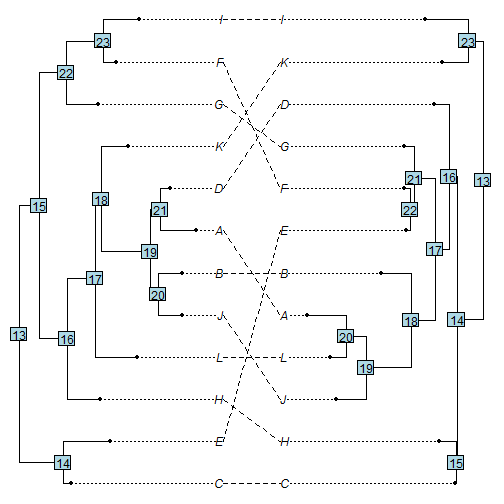We can also change the values represented, of course. For instance:

``````library(stringr)
n.left<-vector()
for(i in 1:obj\$trees[]\$Nnode){
ii<-getDescendants(obj\$trees[],i+Ntip(obj\$trees[]))
n.left[i]<-str_to_lower(paste(sort(obj\$trees[]\$tip.label[ii[ii<=Ntip(obj\$trees[])]]),
collapse=""))
}
n.right<-vector()
for(i in 1:obj\$trees[]\$Nnode){
ii<-getDescendants(obj\$trees[],i+Ntip(obj\$trees[]))
n.right[i]<-str_to_lower(paste(sort(obj\$trees[]\$tip.label[ii[ii<=Ntip(obj\$trees[])]]),
collapse=""))
}
plot(obj)
nodelabels.cophylo(n.left,cex=0.9,bg="white",srt=-90)
nodelabels.cophylo(n.right,which="right",cex=0.9,bg="white",srt=-90)
``````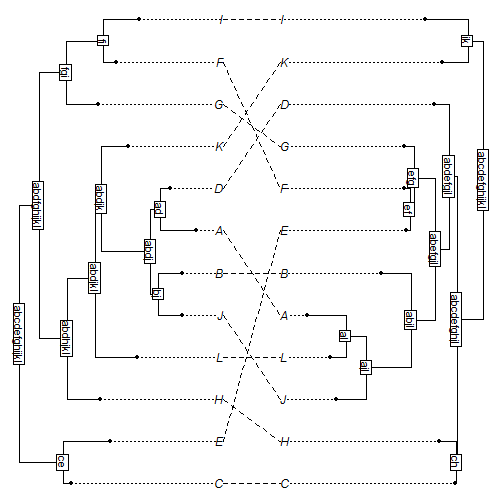Functions to label edges & tips also work for this object class, for instance:

``````plot(obj)
## show rounded edge lengths:
edgelabels.cophylo(round(obj\$trees[]\$edge.length,2),cex=0.7,
edgelabels.cophylo(round(obj\$trees[]\$edge.length,2),cex=0.7,
``````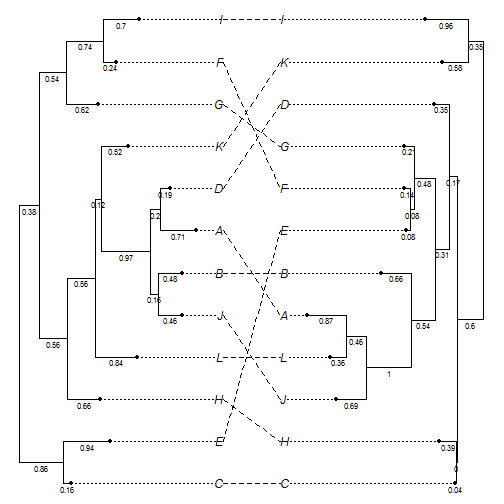``````plot(obj,ftype="off")
tiplabels.cophylo(pch=21,frame="none",bg="grey",cex=1.5)
tiplabels.cophylo(pch=21,frame="none",bg="grey",which="right",cex=1.5)
``````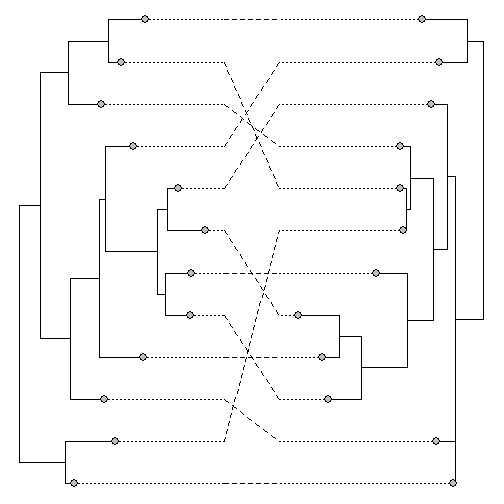We can use any of the options available from `nodelabels` and its sister functions. So, for instance, if we want to overlay reconstructed ancestral states for a discrete character, we can do so easily:

``````## tip data
x
``````
``````##   C   E   H   L   J   B   A   D   K   G   F   I
## "b" "a" "b" "b" "b" "b" "b" "a" "a" "a" "b" "a"
``````
``````y
``````
``````##   H   C   A   L   J   B   F   E   G   D   I   K
## "a" "a" "b" "b" "a" "b" "a" "a" "a" "b" "b" "b"
``````
``````fit.x<-ace(x,obj\$trees[],type="discrete")
fit.y<-ace(y,obj\$trees[],type="discrete")
## plot pies
plot(obj)
nodelabels.cophylo(pie=fit.x\$lik.anc,piecol=c("grey","white"),cex=0.7)
nodelabels.cophylo(pie=fit.y\$lik.anc,piecol=c("grey","white"),cex=0.7,
which="right")
tiplabels.cophylo(pie=to.matrix(x[obj\$trees[]\$tip.label],c("a","b")),
piecol=c("grey","white"),cex=0.4)
tiplabels.cophylo(pie=to.matrix(y[obj\$trees[]\$tip.label],c("a","b")),
piecol=c("grey","white"),cex=0.4,which="right")
``````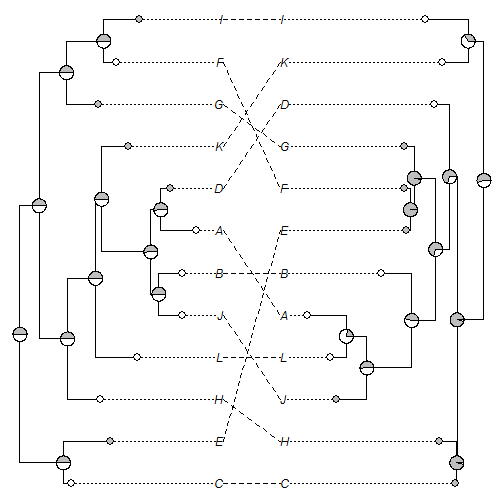Something very important to keep in mind is that the order of the tips, nodes, & edges in the object of class `"cophylo"` may be different than it was in the input trees, particulary if we set `rotate=TRUE` when we made the object.

That's it!

1.This comment has been removed by the author.

2.I needed to use cophyl to do an awesome plot one nuclear data and one chloroplast data 135 tips. I started with RAXML bootstrap trees (bipartition files).
#I want the tips to line up and depth to be to be good enough to show nodes separately
chronopl(nucTree, lambda=0.1)->nucTree
chronopl(cTree, lambda=0.1)->cTree
#plot cophylo with supports
obj<-cophylo(cTree,nucTree) #default optimization worked best for me
plot(obj, fsize=0.3)
nodelabels.cophylo(obj\$trees[]\$node.label, frame="none",cex=0.3)
nodelabels.cophylo(obj\$trees[]\$node.label, frame="none",cex=0.3, which="right")
#this was pretty cool but I wanted to SET UP FOR COLOR coded dots at nodes as numbers are tedious to read
obj\$trees[]\$node.label<-as.numeric(obj\$trees[]\$node.label) #oddly support labels were stored as characters so change to numeric
obj\$trees[]\$node.label<-as.numeric(obj\$trees[]\$node.label)
#set up empty object to store color names for nodes
p1<-character(length(obj\$trees[]\$node.label))
p2<-character(length(obj\$trees[]\$node.label))
#set up ranges that correspond to node support levels
p1[obj\$trees[]\$node.label >= 95] <- "red"
p1[obj\$trees[]\$node.label < 95 & obj\$trees[]\$node.label >= 75] <- "orange"
p1[obj\$trees[]\$node.label < 75] <- "grey"
p1[is.na(obj\$trees[]\$node.label)] <- "white"
p2[obj\$trees[]\$node.label >= 95] <- "red"
p2[obj\$trees[]\$node.label < 95 & obj\$trees[]\$node.label >= 75] <- "orange"
p2[obj\$trees[]\$node.label < 75] <- "grey"
p2[is.na(obj\$trees[]\$node.label)] <- "white" #root node did not have a support value
#check it worked
obj\$trees[]\$node.label
p1
obj\$trees[]\$node.label
p2
#write to single page pdf
pdf(file="support_cophylo_CP_NUC.pdf", 8.5, 11)
plot(obj, fsize=0.3)
nodelabels.cophylo(pch=21,bg=p1,cex=0.7)
nodelabels.cophylo(pch=21,bg=p2,cex=0.7, which="right")
dev.off()

3.This comment has been removed by the author.

4.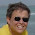This comment has been removed by the author.

5.Dear Liam,

In my input tree I have set the tips to represent distinct diets, but when I use the "tiplabels.cophylo" it doesn't follow the correct classification, as you warned us. I was wondering how can I make the tips to match with the new reordered tree in the object of class "cophylo"? Thanks

1.Dear Anderson.
I think in your case it might be fairly easy. For instance if your diets are in two vectors (diet1 & diet2, for the two trees), you could imagine just sorting each vector by the order of the tips in the reordered trees, e.g.:

obj<-cophylo(...) ## in which ... are the arguments for
## cophylo
diet1<-diet1[trees[]\$tip.label]
diet2<-diet2[trees[]\$tip.label]
tiplabels.cophylo(diet1,...,which="left")
tiplabels.cophylo(diet2,...,which="right")

Or if you're diet is in matrix format:

diet1<-diet1[trees[]\$tip.label,]
diet2<-diet2[trees[]\$tip.label,]

and then the same thing.

2.Thank you very much, Lian. Just one more question, in case I want to change the lines to represent distinct diets connecting the two trees. For example, frugivory species with green lines and herbivory species with blue. Do I need to create different 'assoc' and overlay the plots?

Note: due to the very large amount of spam, all comments are now automatically submitted for moderation.